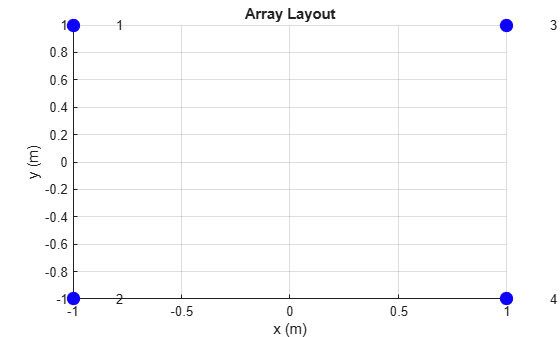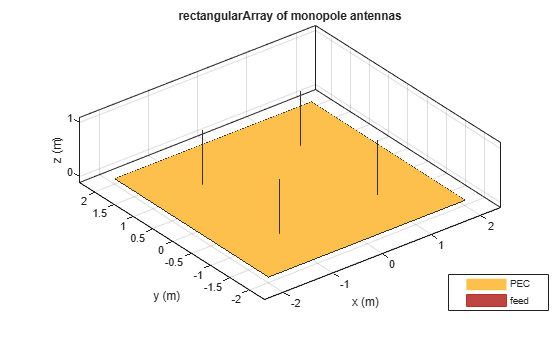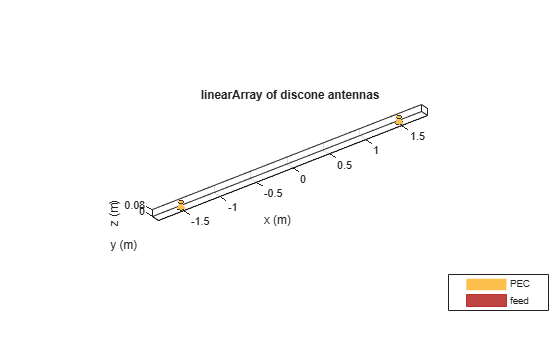rectangularArray

Create rectangular antenna array

Description

The rectangularArray class creates a rectangular antenna array in the X-Y plane. By default, the rectangular array is a four-element dipole array in a 2 x 2 rectangular lattice. The dipoles are center-fed. Each dipole resonates at 70 MHz when isolated.Creation

Description

example

array = rectangularArray creates a rectangular antenna array in the X-Y plane.

example

array = rectangularArray(Name,Value) creates a rectangular antenna array, with additional properties specified by one or more name-value pair arguments. Name is the property name and Value is the corresponding value. You can specify several name-value pair arguments in any order as Name1, Value1, ..., NameN, ValueN. Properties not specified retain default values.

Output Arguments

expand all

Rectangular array, returned as an rectangularArray object.

Properties

expand all

Antenna elements or linear arrays, specified as an antenna or array object.

Example: 'Element',monopole

Number of antenna elements in row and column of array, specified as a two-element vector.

Example: 'Size',[4 4]

Row spacing between two antenna elements, specified as a scalar or vector in meters. By default, the antenna elements are spaced 2m apart.

Example: 'RowSpacing',[5 6]

Data Types: double

Column spacing between two antenna elements, specified as a scalar or vector in meters. By default, the antenna elements are spaced 2m apart.

Example: 'ColumnSpacing',[3 4]

Data Types: double

Antenna elements spatial arrangement, specified as a text input.

Example: 'Lattice',"Triangular"

Data Types: char | string

Excitation amplitude of antenna elements, specified as a scalar or vector. Set the property value to 0 to model dead elements.

Example: 'AmplitudeTaper',3

Data Types: double

Phase shift for antenna elements, specified as a scalar or vector in degrees.

Example: 'PhaseShift',[3 3 0 0]

Data Types: double

Tilt angle of the array specified as a scalar or vector with each element unit in degrees. For more information, see Rotate Antennas and Arrays.

Example: 'Tilt',90,

Example: 'Tilt',[90 90],'TiltAxis',[0 1 0;0 1 1] tilts the array at 90 degrees about the two axes, defined by vectors.

Data Types: double

Tilt axis of the array, specified as:

• Three-element vectors of Cartesian coordinates in meters. In this case, each vector starts at the origin and lies along the specified points on the X-, Y-, and Z-axes.

• Two points in space, each specified as three-element vectors of Cartesian coordinates. In this case, the array rotates around the line joining the two points in space.

• A string input describing simple rotations around one of the principal axes, 'X', 'Y', or 'Z'.

Example: 'TiltAxis',[0 1 0]

Example: 'TiltAxis',[0 0 0;0 1 0]

Example: array.TiltAxis = 'Z'

Data Types: double

Object Functions

 show Display antenna or array structure; display shape as filled patch info Display information about antenna or array beamwidth Beamwidth of antenna charge Charge distribution on metal or dielectric antenna or array surface correlation Correlation coefficient between two antennas in array current Current distribution on metal or dielectric antenna or array surface design Design prototype antenna or arrays for resonance around specified frequency efficiency Radiation efficiency of antenna EHfields Electric and magnetic fields of antennas; Embedded electric and magnetic fields of antenna element in arrays impedance Input impedance of antenna; scan impedance of array layout Display array or PCB stack layout mesh Mesh properties of metal or dielectric antenna or array structure meshconfig Change mesh mode of antenna structure optimize Optimize antenna or array using SADEA optimizer pattern Radiation pattern and phase of antenna or array; Embedded pattern of antenna element in array patternAzimuth Azimuth pattern of antenna or array patternElevation Elevation pattern of antenna or array rcs Calculate and plot radar cross section (RCS) of platform, antenna, or array returnLoss Return loss of antenna; scan return loss of array sparameters Calculate S-parameter for antenna and antenna array objects

Examples

collapse all

Create and plot the layout of a rectangular array of four dipoles.

ra = rectangularArray;
ra.Size = [2 2];
layout(ra);Calculate the scan impedance of a 2x2 rectangular array of dipoles at 70 MHz.

h = rectangularArray('Size',[2 2]);
Z = impedance(h,70e6)
Z = 1×4 complex

26.3455 -56.8671i  26.3401 -56.8729i  26.3455 -56.8671i  26.3401 -56.8729i

Create a rectangular array of monopoles.

m1 = monopole;
mra = rectangularArray('Element',m1);
show(mra);Create an array of discones with element spacing of 3 m.

la = linearArray('Element',discone);
la.ElementSpacing = 3;
show(la)Create a rectangular of the linear array.

ra = rectangularArray("Element",la)
ra =
rectangularArray with properties:

Element: [1x1 linearArray]
Size: [2 2]
RowSpacing: 2
ColumnSpacing: 2
Lattice: 'Rectangular'
AmplitudeTaper: 1
PhaseShift: 0
Tilt: 0
TiltAxis: [1 0 0]

show(ra)Balanis, C.A. Antenna Theory. Analysis and Design, 3rd Ed. New York: Wiley, 2005.